Function Repository Resource:

# PolynomialIdealDimension

Compute the dimension of a polynomial ideal

Contributed by: Daniel Lichtblau
 ResourceFunction["PolynomialIdealDimension"][polys,vars] computes the dimension of the ideal defined by polys in the polynomial ring ℚ[vars].

## Details and Options

PolynomialIdealDimension computes the Krull dimension of an ideal.
PolynomialIdealDimension supports a Modulus option (default 0). If nonzero, the modulus p must be prime. It will regard the polynomials as belonging to the ring p[vars].
Unknowns in the input polynomials that are not specified in vars are regarded as parameters, that is, they lie in the coefficient field.
An ideal containing 1 is considered to have dimension -1.

## Examples

### Basic Examples (3)

Find the dimension of an ideal defined by three polynomials in five variables:

 In:=Out=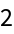When we remove the first variable and regard it as a coefficient parameter the dimension decreases by 1:

 In:=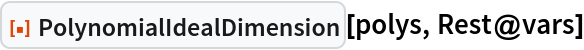Out=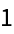If instead we remove the first polynomial then the dimension increases by 1:

 In:=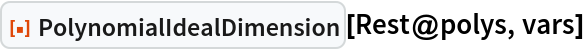Out=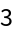### Scope (3)

Find an ideal dimension:

 In:=Out=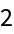Provide a nonzero modulus:

 In:=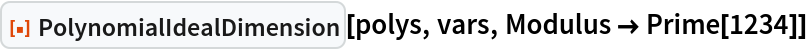Out=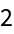For most prime moduli the dimension is the same as in the case of working over the rationals, but there can be finitely many exceptions:

 In:=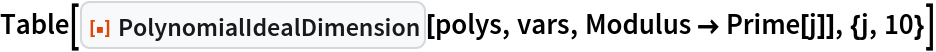Out=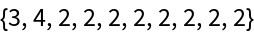### Properties and Relations (4)

Square systems typically have dimension zero; we show this in particular for the Cassou-Nouges benchmark polynomial ideal:

 In:=Out=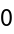Adding a polynomial makes the system overdetermined, so the dimension becomes -1:

 In:=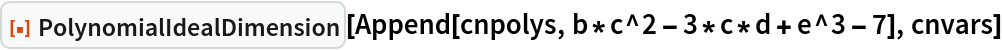Out=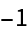Here is a benchmark example from an ISSAC 2001 conference paper with more polynomials than variables; it arose in the context of locating all singular points on a particular algebraic curve:

 In:=Such a polynomial ideal can still have dimension 0 rather than -1; we use a nonzero modulus for speed:

 In:=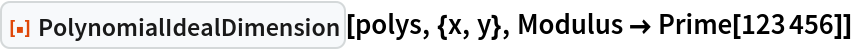Out=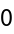### Possible Issues

As with any computation that requires computing a Gröbner basis, this function can be slow.

## Version History

• 1.0.0 – 08 May 2023